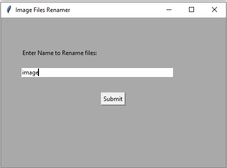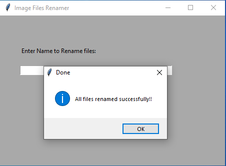# Rename a folder of images using Tkinter

Prerequisites: Python GUI – tkinter, os.listdir() method, os.rename() method

In this article, the task is to rename a batch of images using Python. Now say given n images in a folder having random names. For example, consider the images below.Now the requirement is to rename them in ordered fashion and appending their original dimensions of respective image like image-0-362×326, image-1-351×414, …and so on. Doing this manually would be a tedious task but this target can be achieved using the rename() and listdir() methods in os module.

### rename() method

In Python 3, rename() method is used to rename a file or directory. This method is a part of the os module and comes in extremely handy.

Syntax: os.rename(src, dst)
Parameters:
src: It is the source address of the file to be renamed
dst: It is the destination with the new name.

### listdir() method

The listdir method lists out all the content of a given directory.

Syntax: list = os.listdir(‘Src’)
Parameters:
Src: It is the source to be listed out

The following code will do the job for us. Using the Tkinter module it will ask for the folder path in which all the images are stored. It traverses through the lists of all the images in xyz folder, defines the destination (dst), and source (src) addresses, and renames using rename() method.

Below is the implementation.

## Python3

 `# Python 3 code to rename multiple image` `# files in a directory or folder `     `import` `os  ` `from` `tkinter ``import` `messagebox` `import` `cv2` `from` `tkinter ``import` `filedialog` `from` `tkinter ``import` `*`     `height1 ``=` `0` `width1 ``=` `0`   `# Function to select folder to rename images` `def` `get_folder_path():` `    `  `    ``root ``=` `Tk()` `    ``root.withdraw()` `    ``folder_selected ``=` `filedialog.askdirectory()` `    `  `    ``return` `folder_selected`     `# Function to rename multiple files` `def` `submit():` `    `  `    ``source ``=` `src_dir.get()` `    ``src_dir.``set``("")` `    ``global` `width1` `    ``global` `height1` `    `  `    ``input_folder ``=` `get_folder_path()` `    ``i ``=` `0` `    `  `    ``for` `img_file ``in` `os.listdir(input_folder):`   `        ``file_name ``=` `os.path.splitext(img_file)[``0``]` `        ``extension ``=` `os.path.splitext(img_file)[``1``]`   `        ``if` `extension ``=``=` `'.jpg'``:` `            ``src ``=` `os.path.join(input_folder, img_file)` `            ``img ``=` `cv2.imread(src)` `            ``h, w, c ``=` `img.shape` `            ``dst ``=` `source ``+` `'-'` `+` `str``(i) ``+` `'-'` `+` `str``(w) ``+` `"x" ``+` `str``(h) ``+` `".jpg"` `            ``dst ``=` `os.path.join(input_folder, dst)`   `            ``# rename() function will rename all the files` `            ``os.rename(src, dst)` `            ``i ``+``=` `1` `            `  `    ``messagebox.showinfo("Done", "``All` `files renamed successfully !!")`       `# Driver Code` `if` `__name__ ``=``=` `'__main__'``:` `    ``top ``=` `Tk()` `    ``top.geometry("``450x300``")` `    ``top.title("Image Files Renamer")` `    ``top.configure(background ``=``"Dark grey")`   `    ``# For Input Label` `    ``input_path ``=` `Label(top, ` `                       ``text ``=``"Enter Name to Rename files:",` `                       ``bg ``=``"Dark grey").place(x ``=` `40``, y ``=` `60``)` `    `  `    ``# For Input Textbox` `    ``src_dir ``=` `StringVar()` `    ``input_path_entry_area ``=` `Entry(top,` `                                  ``textvariable ``=` `src_dir,` `                                  ``width ``=` `50``).place(x ``=` `40``, y ``=` `100``)`   `     ``# For submit button` `    ``submit_button ``=` `Button(top, ` `                           ``text ``=``"Submit",` `                           ``command ``=` `submit).place(x ``=` `200``, y ``=` `150``)`   `    ``top.mainloop()`

Output :Whether you're preparing for your first job interview or aiming to upskill in this ever-evolving tech landscape, GeeksforGeeks Courses are your key to success. We provide top-quality content at affordable prices, all geared towards accelerating your growth in a time-bound manner. Join the millions we've already empowered, and we're here to do the same for you. Don't miss out - check it out now!